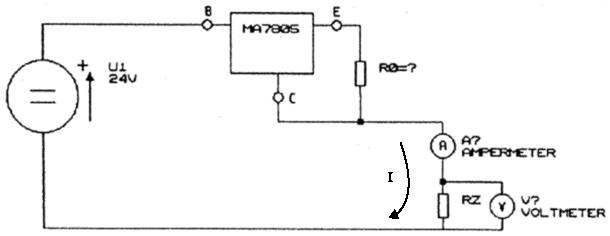# MA7805 as a Voltage and Current Source

#### Introduction:

The goal of this task is to

• exercise the technique of measurement of voltage and current in an electrical circuit, and the measurement of impedance of a source,
• verify the difference between the characteristics of voltage and current sources in practice,
• understand the block scheme of a regulation circuit with feedback.

#### Instructions:

1. Draw a block scheme of integrated voltage stabilizer MA7805 (without the circuits of temperature and current protection) explaining the principle of its operation.
2. Familiarize yourself with the limits of the circuit's parameters in the data sheet of the device and write the limits, that are essential for the realization of this task, to the protocol.
3. Prove the functionality of the voltage stabilizer in the standard scheme for load currents of 5 mA, 10 mA, 50 mA and 300-600 mA (that means to measure the dependence of the output voltage on the load current). Bring out the scheme in the protocol and determine the internal resistance of this implementation of voltage stabilizer.
4. Measure the dependence of the current in quiescence I(0) (current flowing through the circuit between the outlets B and C, having the E not connected) on the supply voltage U(BC) and determine the minimal voltage U(BC) necessary to operate the circuit as a voltage source. Bring out the used scheme in the protocol.
5. Propose the resistor R0 in the scheme at Figure 1 so that the circuit will operate as a current source giving current of 20 mA. Explain the function of this scheme and bring out the equation for the current I flowing to the load Rz.
6. Prove by measurement that the circuit operates as a current source. Draw graph of dependence of the voltage on the value of the resistor Rz. Bring out the range of values of the load Rz allowing the circuit to operate as a current source and explain the limitations.Figure 1: Scheme with MA7805 as a current source

Josef Blažej - contact - blazej   troja.fjfi.cvut.cz - phone: +420 224 358 659
Czech Technical University in Prague - Faculty of Nuclear Sciences and Physical Engineering
Brehova 7, 115 19 Prague 1, Czech Republic